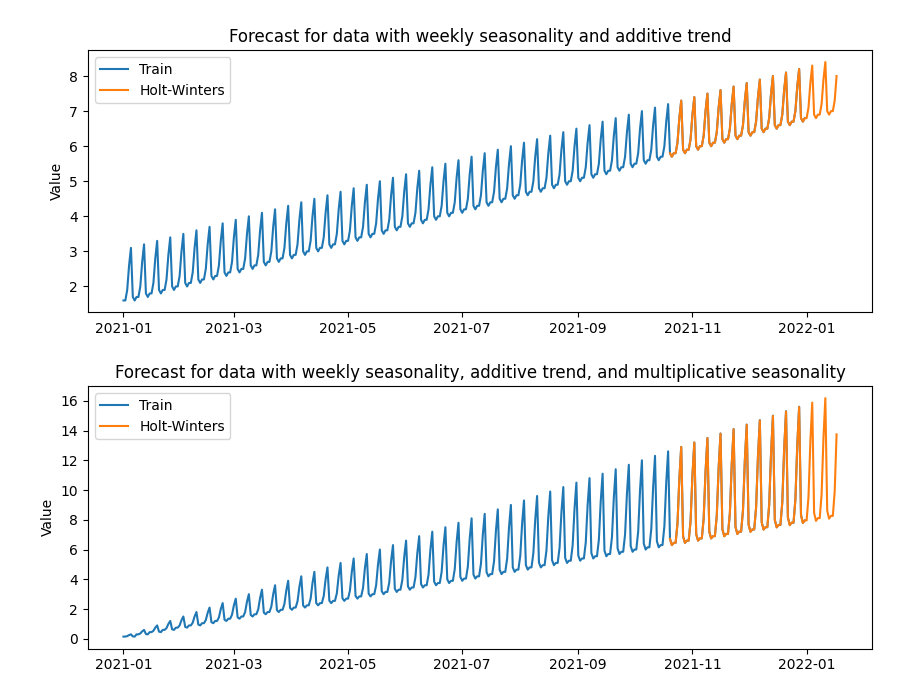# Holt-Winters Predictor

For the Holt-Winters example we use forged daily data with a weekly seasonality. We predict two types of data, the first dataset displays an additive trend and an additive seasonality, and the second dataset displays an additive trend and a multiplicative seasonality.```import os
import warnings

import matplotlib.pyplot as plt
import pandas as pd

from indsl.forecast.holt_winters_predictor import holt_winters_predictor as hwp

# suppress "No frequency information was given" warning - Frequency information is derived from datetime index
warnings.filterwarnings("ignore")

base_path = "" if __name__ == "__main__" else os.path.dirname(__file__)
data = pd.read_csv(os.path.join(base_path, "../../datasets/data/seasonal_with_trend_data.csv"), sep=";", index_col=0)
data.index = pd.to_datetime(data.index)

# calculate the forecast for both data types
multiplicative_res = hwp(data["multiplicative"], seasonal_periods=7, seasonality="mul", steps=90)

# plot result
fig, ax = plt.subplots(2, 1, figsize=[9, 7])
ax.set_ylabel("Value")
ax.set_title("Forecast for data with weekly seasonality and additive trend")

ax.plot(data.index, data["multiplicative"], label="Train")
ax.plot(multiplicative_res.index, multiplicative_res, label="Holt-Winters")
ax.set_title("Forecast for data with weekly seasonality, additive trend, and multiplicative seasonality")
ax.set_ylabel("Value")

_ = ax.legend(loc=0)
_ = ax.legend(loc=0)